Dipole moment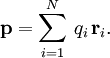$\mathbf{p} = \sum_{i=1}^N \, q_i \, \mathbf{r}_i.$
For molecular systems, it is customarily given in units of Debyes, after the physicist Peter J. W. Debye. In SI units,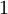$1$D equals approximately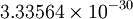$3.33564\times 10^{-30}$ Coulomb-meter (exactly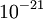$10^{-21}$C m$^2$/s divided by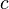$c$, the speed of light in vacuum). Conversely, 1 C m =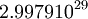$2.9979 10^{29}$D. This is unit is convenient for molecular systems since 1Å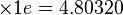$\times 1 e=4.80320$D.